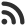Feed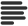Articles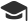Tutorials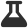Lab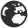CompaniesLeaderboard
 DG Student at BIT Mesra April 12, 2020, 12:37 p.m. ⋅ 1509 views

# Find all the prime numbers of given number of digits

To find the number of a given number of digits we will use Sieve of Eratosthenes.  We will find all the numbers in range of given number of digits, then we'll filter out prime numbers from it. For example, range for 1 digit would be 1 to 9. Similarly, range for 2 digit would be 10 to 99 and primes in 1 digit range would be 2,3,5 and 7.

Problem

Given a digit D, find all the prime numbers of D digits.

Example

``````D = 1

Prime no = 2 3 5 7

D = 2

Prime no = 11 13 17 19 23 29 31 37 41 43 47 53 61 67 71 73 79 83 89 97``````

Solution

As we know, all the numbers of D digits lie in range  [10(D – 1), 10D – 1]

All, we have to do now is to filter out prime numbers from that range and print them all. You can use nieve approach to iterate over the enitre range and check if the number is prime, if it is you'll print it otherwise ignore it.

An efficient solution would be using Sieve of Eratosthenes.  Sieve of Eratosthenes is used to find prime numbers in a given range.

Implementation

``````// C++ implementation of the approach
#include <bits/stdc++.h>
using namespace std;

const int sz = 1e5;
bool isPrime[sz + 1];

// generate a list of primes before hand using sieve or ertst.
void sieve()
{
memset(isPrime, true, sizeof(isPrime)); // initally assume all prime

isPrime = isPrime = false;  // mark 0 and 1 as not prime

for (int i = 2; i * i <= sz; i++) {
if (isPrime[i]) {
for (int j = i * i; j < sz; j += i) {
isPrime[j] = false;  // if prime is found all of its multiples are not prime, mark them all
}
}
}
}

void solve(int d)
{
// setting range
int lower = pow(10, d - 1);
int higher = pow(10, d) - 1;

// iterate over range and get its primarility from sieve we created above.
for (int i = lower; i <= higher; i++) {
if (isPrime[i]) {
cout << i << " ";
}
}
}

int main()
{
sieve();  // initialize sieve
int d = 2;
solve(d);

return 0;
}
``````
``11 13 17 19 23 29 31 37 41 43 47 53 59 61 67 71 73 79 83 89 97``

Events

Nov. 28, 2018, 5:30 p.m.

Python from zero to hero

place Delhi

Aug. 13, 2018, 5:30 p.m.

Python from zero to hero

place Bangalore ( HackersFriend office BTM Layout)